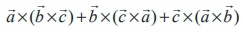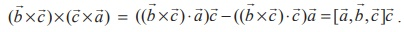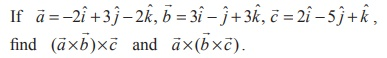Home | | Maths 12th Std | Jacobi’s Identity and Lagrange’s Identity

# Jacobi’s Identity and Lagrange’s Identity

Jacobi’s Identity Theorem and Lagrange’s Identity Theorem : Definition, Theorem, Proof, Solved Example Problems, Solution

Jacobi’s Identity and Lagrange’s Identity

Theorem 6.9 (Jacobi’s identity)

For any three vectors, we have=.

Proof

Using vector triple product expansion, we haveAdding the above equations and using the scalar product of two vectors is commutative, we get.

Theorem 6.10 (Lagrange’s identity)Proof

Since dot and cross can be interchanged in a scalar product, we getExample 6.19

Prove thatSolution

Using the definition of the scalar triple product, we get..............(1)

By treating (×) as the first vector in the vector triple product, we findUsing this value in (1), we getExample 6.20

Prove that.

Solution

Treating (×) as the first vector on the right hand side of the given equation and using the vector triple product expansion, we getExample 6.21

For any four vectors, we haveSolution

Taking= (×)  as a single vector and  using the vector triple product expansion, we getExample 6.22State whether they are equal.

SolutionExample 6.23Solution (i)

By definition,On the other hand, we haveTherefore, from equations (1) and (2), identity (i) is verified.

The verification of identity (ii) is left as an exercise to the reader.

Tags : Definition, Theorem, Proof, Solved Example Problems, Solution , 12th Mathematics : UNIT 6 : Applications of Vector Algebra
Study Material, Lecturing Notes, Assignment, Reference, Wiki description explanation, brief detail
12th Mathematics : UNIT 6 : Applications of Vector Algebra : Jacobi’s Identity and Lagrange’s Identity | Definition, Theorem, Proof, Solved Example Problems, Solution